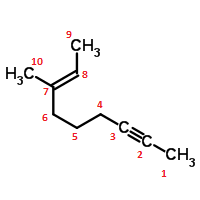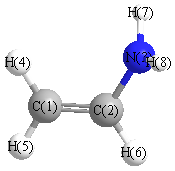# Exercise 10

$$\newcommand{\vecs}{\overset { \rightharpoonup} {\mathbf{#1}} }$$ $$\newcommand{\vecd}{\overset{-\!-\!\rightharpoonup}{\vphantom{a}\smash {#1}}}$$$$\newcommand{\id}{\mathrm{id}}$$ $$\newcommand{\Span}{\mathrm{span}}$$ $$\newcommand{\kernel}{\mathrm{null}\,}$$ $$\newcommand{\range}{\mathrm{range}\,}$$ $$\newcommand{\RealPart}{\mathrm{Re}}$$ $$\newcommand{\ImaginaryPart}{\mathrm{Im}}$$ $$\newcommand{\Argument}{\mathrm{Arg}}$$ $$\newcommand{\norm}{\| #1 \|}$$ $$\newcommand{\inner}{\langle #1, #2 \rangle}$$ $$\newcommand{\Span}{\mathrm{span}}$$ $$\newcommand{\id}{\mathrm{id}}$$ $$\newcommand{\Span}{\mathrm{span}}$$ $$\newcommand{\kernel}{\mathrm{null}\,}$$ $$\newcommand{\range}{\mathrm{range}\,}$$ $$\newcommand{\RealPart}{\mathrm{Re}}$$ $$\newcommand{\ImaginaryPart}{\mathrm{Im}}$$ $$\newcommand{\Argument}{\mathrm{Arg}}$$ $$\newcommand{\norm}{\| #1 \|}$$ $$\newcommand{\inner}{\langle #1, #2 \rangle}$$ $$\newcommand{\Span}{\mathrm{span}}$$

Solutions, hints and answers can be found in the Solutions Page. It is strongly recommended that students do not look at the solutions until after completing or attempting to complete the problems. Going straight to the solutions will reduce the point of the exercises.

## Q1

The molecular orbital diagram for the carbide ion ($$C_2^{2-}$$) would show which of the following molecular orbitals?

1. $$\sigma_{1s}^2 {\sigma^*_{1s}}^2 \sigma_{2s}^2 {\sigma*_{2s}}^2 \pi_{2pp}^4$$
2. $$\sigma_{1s}^2 {\sigma^*_{1s}}^2 \sigma_{2s}^2 {\sigma^*_{2s}}^2 \pi_{2pp}^4 \sigma_{2p}^2$$
3. $$\sigma_{1s}^2 {\sigma^*_{1s}}^2 \sigma_{2s}^2 {\sigma^*_{2s}}^2 \pi_{2pp}^4 \sigma_{2p}^2 {\pi^*_{2p}}^2$$
4. $$\sigma_{1s}^2 {\sigma^*_{1s}}^2 \sigma_{2s}^2 {\sigma^*_{2s}}^2 \pi_{2pp}^4 \sigma_{2p}^2 {\pi^*_{2p}}^4$$

## Q2

What is the hybridization of the sulfur atom is $$SF_4$$?

1. $$sp^2$$
2. $$sp^3$$
3. $$sp^3d$$
4. $$sp^3d^2$$

## Q3

What is the hybridization of the oxygen atom in water?## Q4

When a double bond is formed between two atoms, one of the bonds is a sigma bond and the other is a pi bond. The pi bond is created by the overlap of...

## Q5

The valence bond hybrid atomic orbitals $$sp^3$$ are used by both $$C$$ in $$CH_4$$ and $$O$$ in $$H_2O$$. Yet, the bond angles between atoms in $$H_2O$$ are less than in $$CH_4$$. Explain.

## Q6

Describe completely the main features of each of the following and explain what useful information we gain from each.

1. Lewis Structures
2. Valence Shell Electron Pair Repulsion (VSEPR) theory
3. Valence Bond (VB) theory
4. Molecular Orbital (MO) theory

## Q7

1. Draw all possible resonance Lewis structures for $$NO_3^{-1}$$. Include formal charges and the correct angles.
2. Draw the "realistic" hybrid resonance structure with appropriate angles that takes and average of the Lewis structures in part a. Include formal charges (fractions) and bond orders (fractions). Include nonbonding electrons on central atom but not on terminal atoms.
3. Sketch the valence bond probability picture of one of the $$NO_3^{-1}$$ resonances. Identify and label the hybridized orbitals. Identify $$\sigma$$ and $$\pi$$ bonds.

## Q8

For each of the following: $$B_2$$, $$Ne_2$$, $$O_2$$

1. Give the molecular orbital (MO) energy diagram for each.
2. Write the MO configurations for $$O_2$$ starting with ($$\sigma_{1s})^2$$
3. Give the bond order of each molecule
4. List the species in decreasing order of bond energy and stability
5. Identify each as diamagnetic or paramagnetic?
6. Using the bond order information, which is least expected to exist. Explain why.
7. Which would have the shortest bond length? Explain

## Q9

Consider (2E)-3-Methyl-2-nonen-7-yne, an organic molecule with the molecule skeleton structure below:1. Draw electron-dot structure for this molecule
2. Estimates of bond angles (all of them)
3. Identify the hybridization of all heavy (non-H) atoms
4. Do all the heavy atoms lie in the same plane?
5. What is the description of the bonds formed between the heavy atoms (take into account nature of bond and the hybridization of bonding orbitals). For example: The (C5,C6) single bond is a sigma type bond represented by the overlap of a Carbon sp3 hybridized orbital on C5 with a Carbon sp3 hybridized orbital on C6.

## Q10

Several isomers $$CH_2CHNH_2$$ are possible. Consider two isomers: ethanimine (on left) and vinylamine (on right)1. Draw an acceptable Lewis structure of each isomers.
2. Predict the bond angles of this isomer and give the hybridization of each of the heavy (non-H) atoms.
3. Describe the bonds formed between the heavy atoms. Use the $$\sigma$$ and $$\pi$$ formalism in your description.
4. Compare the carbon-nitrogen bond lengths. Which is longer and why?

## Q11

Answer the following questions for the set of molecules: $$H_2^+$$, $$H_2$$, $$H_2^-$$, $$H_2^{-2}$$.

1. Write down the electronic configuration of each molecule.
2. Arrange the molecules in order of increasing bond length and bond dissociation energy
3. For each molecule, sketch a graph of the electronic energy versus the H-H interatomic distance. The 4 graphs should been scaled identically so that they can be compared with one another.
4. Which of the molecules will be paramagnetic? Also answer questions a-d for the set $$He_2^{2+}$$, $$He_2^+$$, and $$He_2$$.

Exercise 10 is shared under a not declared license and was authored, remixed, and/or curated by LibreTexts.